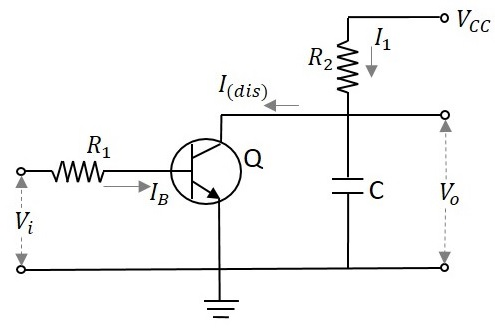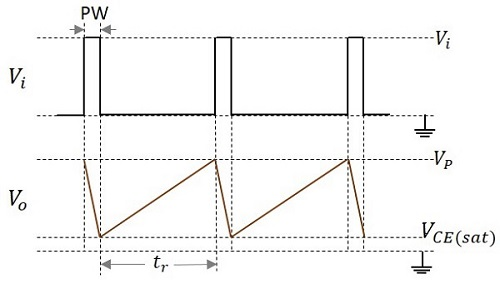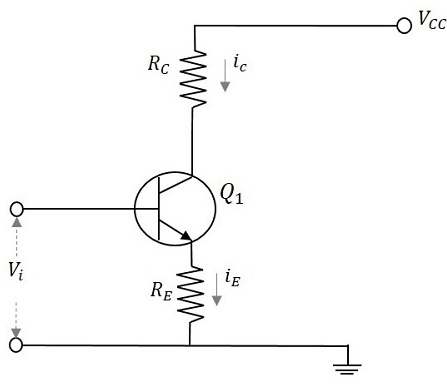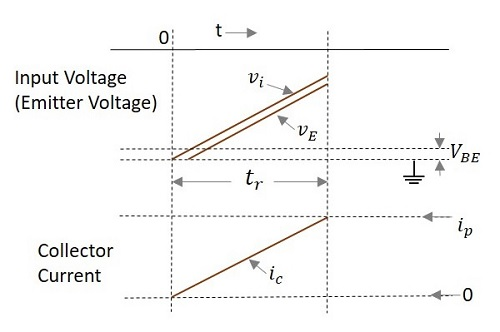# Types of Time Base Generators

As we have an idea that there are two types of time base generators, let us try to know about the basic circuits of those time base generator circuits.

## Voltage Time base Generator

A time base generator that provides an output voltage waveform that varies linearly with time is called as a Voltage Time base Generator.

Let us try to understand the basic voltage time base generator.

### A Simple Voltage Time base Generator

A basic simple RC time base generator or a Ramp generator or a sweep circuit consists of a capacitor C which charges through VCC via a series connected resistor R2. It contains a BJT whose base is connected through the resistor R1. The capacitor charges through the resistor and discharges through the transistor.

The following figure shows a simple RC sweep circuit.By the application of a positive going voltage pulse, the transistor Q turns ON to saturation and the capacitor rapidly discharges through Q and R1 to VCE (sat). When the input pulse ends, Q switches OFF and the capacitor C starts charging and continues to charge until the next input pulse. This process repeats as shown in the waveform below.When the transistor turns ON it provides a low resistance path for the capacitor to discharge quickly. When the transistor is in OFF condition, the capacitor will charge exponentially to the supply voltage VCC, according to the equation

$$V_0 = V_{CC}[1 - exp(-t/RC)]$$

Where

• VO = instantaneous voltage across the capacitor at time t
• VCC = supply voltage
• t = time taken
• R = value of series resistor
• C = value of the capacitor

Let us now try to know about different types of time base generators.

The circuit just we had discussed, is a voltage time base generator circuit as it offers the output in the form of voltage.

## Current Time base Generator

A time base generator that provides an output current waveform that varies linearly with time is called as a Current Time base Generator.

Let us try to understand the basic current time base generator.

### A Simple Current Time base Generator

A basic simple RC time base generator or a Ramp generator or a sweep circuit consists of a common-base configuration transistor and two resistors, having one in emitter and another in collector. The VCC is given to the collector of the transistor. The circuit diagram of a basic ramp current generator is as shown here under.A transistor connected in common-base configuration has its collector current vary linearly with its emitter current. When the emitter current is held constant, the collector current also will be near constant value, except for very smaller values of collector base voltages.

As the input voltage Vi is applied at the base of the transistor, it appears at the emitter which produces the emitter current iE and this increases linearly as Vi increase from zero to its peak value. The collector current increases as the emitter current increases, because iC is closely equal to iE.

The instantaneous value of load current is

$$i_L i_C \thickapprox (v_i - V_{BE})/R_E$$

The input and output waveforms are as shown below.